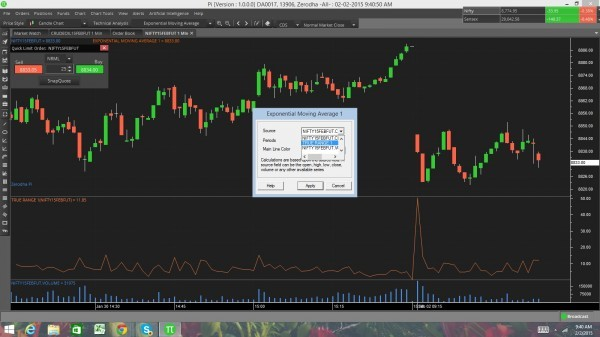# True Range Indicator

In PI software, we have an indicator True Range, I wanted to know whether we can draw a moving average line in True range indicator or do we have a indicator which provides this data, i need a 10 periods moving average of True Range data points to know the volatility??? Please let me know if this is possible?

Yes you can draw a moving average on True range (any indicator actually).

Firstly add true range on the chart.

Once you have added, now add the EMA, but while adding the EMA, choose True range in the drop down, as shown below.Thanks a lot for this information…PI is a very useful tool!

1 Like